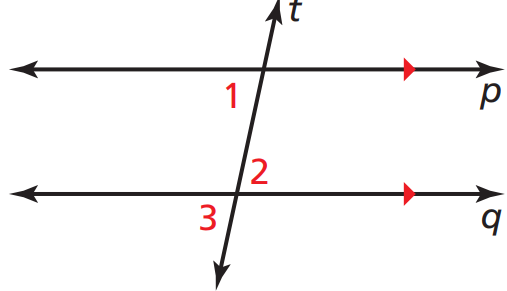# mmathhh

Classified in Mathematics

Written at onEnglish with a size of 51.45 KB.

###### 35 = 7x Subtract 9 from each side. 5 = x Divide each side by 7.Draw a diagram. Label a pair of alternate
interior angles as ∠1 and ∠2. You are looking for
an angle that is related to both ∠1 and ∠2. Notice
that one angle is a vertical angle with ∠2 and a
corresponding angle with ∠1. Label it ∠3.